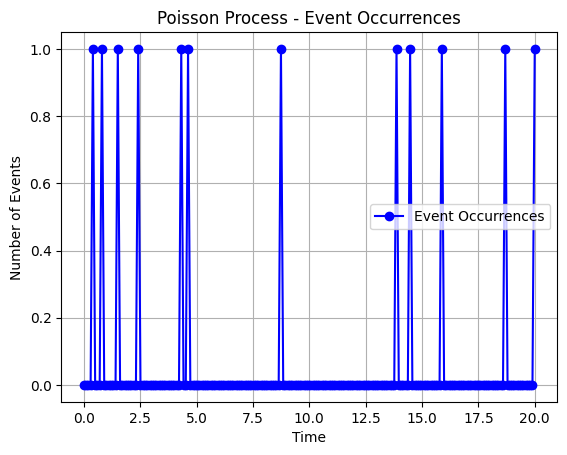Course Content

# Probability Theory Basics

Probability Theory Basics

##Exponential Distribution

The exponential distribution is a continuous probability distribution that models the time between events in a Poisson process.We remember that the Poisson process describes the number of events that occurred during some period of time. On the other hand, exponential distribution describes the time between the occurrence of two successive events (the distance between two adjacent non-zero points in the graph above).
Poisson distribution is parameterized by `mu` parameter, which describes the average number of accidents by the time unit. The exponential distribution is parametrized by the parameter `scale` which determines the average time between two accidents.

Note

There is a clear relationship between the Poisson process parameter `mu` and the exponential distribution parameter `scale`:
`mu` = `1 \ scale` for one unit of time

Let's look at the example.
Suppose the average time between customer arrivals at a store is `5` minutes. What is the probability that the next customer arrives within `3` minutes?

We have a Poisson process where an event is the customer's arrival. The average time between two arrivals is `5` minutes. As a result, we can use exponential distribution to calculate the corresponding probability:We also use `.cdf()` method of the `scipy.stats.expon` class with a specified `scale` parameter to calculate the corresponding probability on the interval `[0,3]`.